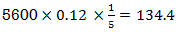Goseeko blog# What is simple interest?

We can lend or borrow money from one entity to another. The price to be paid for the use of a certain amount of money for a certain period of time is known as interest. In other words, We can define it as “Simple interest is a method to calculate the amount of interest charged on a sum at a given rate and for a given period of time. “

The money we borrow or lent is called principal and The interest may be payable yearly, half-yearly, quarterly or monthly.

Calculation of interest is one of the most basic uses of mathematics in finance.

So that, we can define the SI as- “the price has to be paid for the use of a certain amount of money or principal for certain period, is called interest”

## Amount- This is the sum of the principal and the interest at any time.

The rate of intrst is denoted by ‘i’.

Let the principal is 100 Rs. And the interest is 8 then we can say that the rate of interest is 8 percent per annum or we can write it as r = 8%

Suppose ‘P’ is the principal and ‘n’ is the time for which the principal is given and ‘r’ be the rate of interest per annum

‘I’ be the amount of interest

And ‘i’ be the rate of interest per unit

Then-

SI = Principal ×Rate ×Time

## Abbreviations

I: Interest, the amount of money that you pay to borrow money or the amount of money that you earn on a deposit.

P: Principal which is the original sum of money

t: Time duration for which the money is borrowed/ deposited.

r: Rate of interest which is usually expressed as percent that is paid for money borrowed, or earned for money deposited.

We can calculate the amount, so that

## Solved examples

Example: Sushmita invested 5000rs. At the rate of 8 per cent per annum then what will be the value of the amount she invested in 5 years.

Sol:

Here P = 5000, r = 8/100 = 0.08, t = 5, so that

Hence the required amount is – 7000

Example: Find out the SI on 5600 rs. At 12 percent per annum from July 15 to September 26, 2020.

Sol:

Here we calculate the time, so that total days from July 15 to sept 26 = 73 days or 73/365 = 1/5 years

And P = 5600, r = 12/100 = 0.12

SI = P.r.t. =Hence the SI is – 134.40

Example: To buy a new truck, Jack borrowed Rs. 5,00,000 at 8% annual S.I. for 3 years. How much interest will he pay?

Sol:

Here, P = 5,00,000, r = 8/100=0.08, t = 3 years, I =?

So that using the formula I = P × r × t,

SI (I) = 500000 × 0.08 × 3

= Rs. 1,20,000.

Therefore Jack will pay Rs. 1,20,000 as interest for the truck loan.

Example: In how many years will a sum be double of itself at 5% p.a. S.I.?

Sol:

Amount = 2 × Principal

Given r = 0.05, t=?

From A = P + P×r×t,

A = P (1 + rt).

According to question, 2P = P [1 + t(0.05)]

2 = 1 + t (0.05)

t = 1/0.05

= 20

A sum will be double of itself at 5% p.a. SI in 20 years.

nterested in learning about similar topics? Here are a few hand-picked blogs for you!